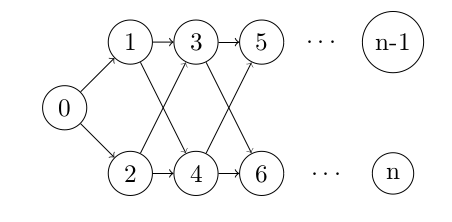Using Topological sort v/s using DFS

Question:

My question is what suggests that we’ve to use topo sort in this question? Why is my following DFS approach incorrect?

double prob[MAX];
int outdegree[MAX];
void DFS(vi adj[], int s, double parent_probability_through_this_path){
for(int i = 0; i < adj[s].size(); i++){
}

}
Full code: https://www.hackerearth.com/submission/33961586/
I am simply adding all possible paths of reaching a node. There may be multiple paths to a node and hence I am not checking the visited condition. When a node is visited again, I simply add the prob of reaching here through this DFS path to the probability that existed by already visiting it through some other DFS path.This is only passing a few TCs.

PS: vi is vector. Calling function like this
prob[r] = 1;